# Euclidean plane

## Definition

The Euclidean plane, denoted$\R^2$, is defined as the product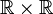$\R \times \R$, i.e., the set of ordered pairs of real numbers. It is equipped with the product topology from the Euclidean topology on the real line. In addition to a topological structure, the Euclidean plane also has a natural metric structure, group structure, and other structures, all of them giving rise to the same topology.

The Euclidean plane is a special case of Euclidean space$\R^n$ with the parameter value$n = 2$.

## Equivalent spaces

Space How it is equivalent to the Euclidean plane geometrically
open circular disk in$\R^2$, i.e., the set of all points at distance less than a fixed positive number from a fixed point (interior region of a circle), e.g., the set$\{(x,y) : x^2 + y^2 < 1 \}$ In polar coordinates, do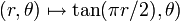$(r,\theta) \mapsto \tan(\pi r/2), \theta)$
complex numbers$\mathbb{C}$ under the topology arising from the modulus metric Identify a complex number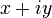$x + iy$ with the ordered pair$(x,y)$; here, the modulus becomes the Euclidean distance between points.
interior of a bounded rectangle, e.g.,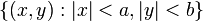$\{ (x,y) : |x| < a, |y| < b \}$ where$a,b$ are positive reals The homeomorphism$(x,y) \mapsto (\tan(\pi x/(2a)), \tan(\pi y/(2b))$
2-sphere minus a point on it Stereographic projection
Right circular cylinder minus a line on it parallel to the axis of the cylinder

## Algebraic topology

The Euclidean plane is a contractible space, i.e., it has the homotopy type of a point. So its zeroth homology and cohomology groups with coefficients in any module are equal to that module, and all higher homology and cohomology groups are zero.

Its zeroth homotopy set is a one-point set (which can be interpreted as the trivial group), and its fundamental group and all higher homotopy groups are trivial groups.

Some important numerical invariants:

Invariant General description Description of value for Euclidean space
Betti numbers The$k^{th}$ Betti number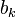$b_k$ is the rank of the$k^{th}$ homology group.$b_0 = 1$, all higher$b_k$ are$0$
Poincare polynomial Generating polynomial for Betti numbers$1$ (the constant polynomial)
Euler characteristic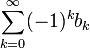$\sum_{k=0}^\infty (-1)^k b_k$ 1 (hence it is a space with Euler characteristic one)

## Algebraic and coalgebraic structure

### Algebraic structure

The Euclidean plane has a natural structure as a topological group, namely, the additive group of the vector space$\R^2$. This is a real Lie group.

It can also be thought of as the additive group of$\mathbb{C}$, making it a complex Lie group.